Let’s explore the world of the eight-sided octagon.Author Taylor HartleyExpert Reviewer

Published: August 24, 2023Let’s explore the world of the eight-sided octagon.Author
Taylor HartleyExpert Reviewer

Published: August 24, 2023Let’s explore the world of the eight-sided octagon.Author
Taylor HartleyExpert Reviewer

Published: August 24, 2023Key takeaways

• An octagon is a shape with 8 eight sides – It’s actually a type of polygon, which is typically a shape with at least three sides.
• There are different types of octagons – While regular octagons follow a distinct set of rules, you may encounter irregular octagons along with “concave” octagons. Don’t worry—we’ll show you what that means!
• Calculating the perimeter of an octagon can be tricky – For a regular octagon, you simply multiply the length of the sides by 8, but for other octagons, you’ll need your addition skills!

Octagons are one of the coolest shapes you’ll learn about as you start to investigate different types of polygons. They might look strange, but they’re easy to understand once you figure out what their main traits are.

Come explore the octagon with us and discover how to identify these unique shapes when you see them out in the wild!

## What is an octagon?Octagons belong to the polygon family of shapes. Polygons are closed plane figures with at least three sides and three angles.

When we say closed plane, we mean the end of each side touches the end of another, creating a closed space or shape. If the sides don’t touch or if there is a gap between the sides, then it is not considered a closed plane.

Triangles, squares, and rectangles are all closed plane figures—and polygons! The octagon is grouped together with these shapes, but is unique in how many sides it has—eight in total!

## What is an octagon’s shape?An octagon has eight sides with eight individual angles. Each of these sides connect to form what is known as a vertex, or the point where two sides meet together to form an angle.

Like we mentioned before, an octagon is considered as a polygon, meaning each of these eight sides create a closed shape or closed plane, with specific angles formed around the points where the sides connect. This shape follows the same rules as a basic triangle or rectangle, but with a few extra sides to boot.

Now, let’s dive into the different types of octagons and the kinds of shapes they can form.

### A regular octagonA regular octagon has eight equal sides and eight equal angles as well. To identify a regular octagon, all its features have to be equal to each other.

That means each of the sides should have an equal length, and all the angles in that octagon should be the same as well. One easy rule to remember is that in a regular octagon, each angle is equal to 135°, and the sum of these eight angles is 1080°.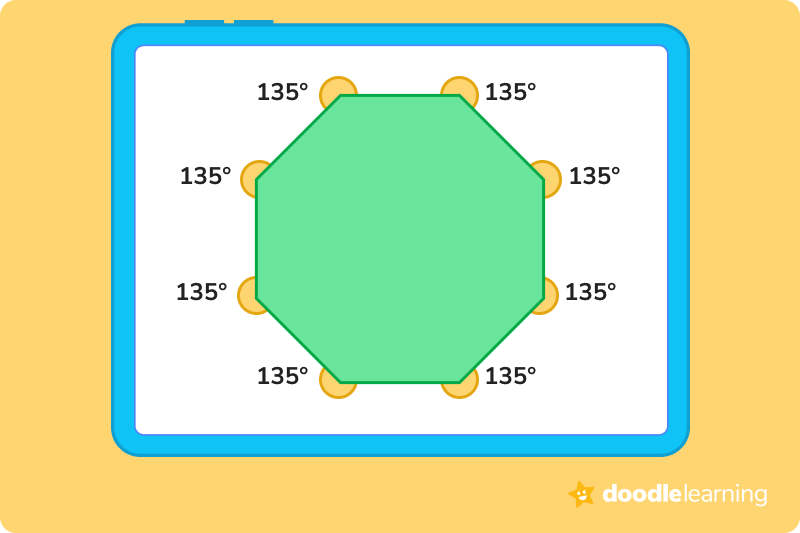A regular octagon has equal sides and angles that each measure 135°.

### An irregular octagonIrregular octagons also have eight sides and angles. However, irregular octagons have sides and angles with unequal values. Not all of an irregular octagon’s sides are equal in length, and their angles aren’t equal either.

But the sum of these angles is still equal to 1080°, just like a regular octagon. Put simply, an irregular octagon has unequal sides and unequal angles, but the sum of these angles equals the same value as a regular octagon.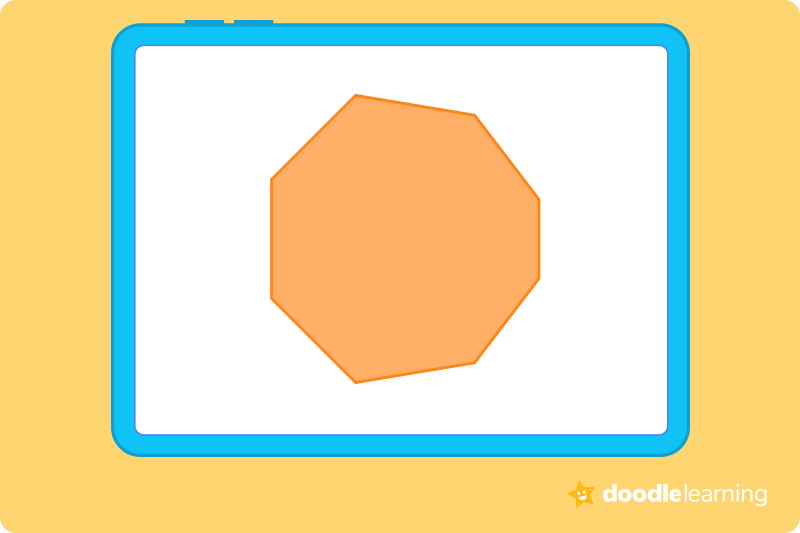An irregular octagon has eight sides, but they are not all of equal length.

## Octagons in real lifeOctagons aren’t the most common thing to see in nature, so don’t go expecting to find them in the flowers in your backyard or in the pepperoni slices on your pizza. However, there are a few man-made designs that use the octagon.

Take a look out your car window, and you will probably see a big red octagon. Stop signs are almost always octagons, at least in the United States!

Many of the umbrellas we use during thunderstorms are also shaped just like an octagon too.

Many of the octagons you see are shaped like a regular octagon, but some irregular octagons are still common in design and fashion as well.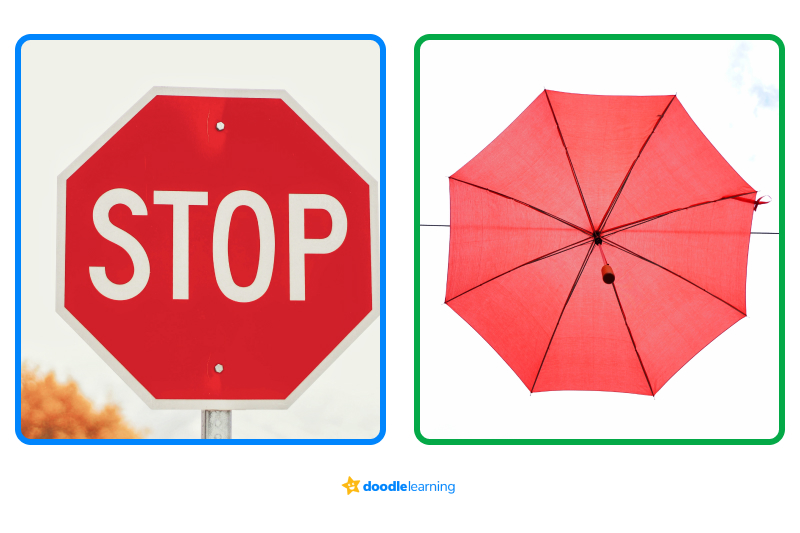## Properties of an octagonLet’s review some of the main properties a shape must have in order to be called an octagon. When we say properties, we’re referring to the specific things a shape needs to be considered a regular octagon.

An octagon must have:

• 8 sides
• 8 angles
• All sides and all angles are equal
• A total of 20 possible diagonals
• Interior angles that equal a total sum of 1080°
• Each interior angle equals 135°
• Exterior angles that equal a total sum of 360°
• Each exterior angle equals 45°

## Other types of octagonsRemember that the two main types of octagons are regular octagons, which follow the attributes above, and irregular octagons, which may not always align with the properties above, but they do have eight sides.

Now we’re going to explore two additional types of octagons: convex and concave octagons.

### Convex octagonWhen a polygon such as an octagon is “convex,” that means none of its vertices are pointed inward. That means that all of the interior angles are less than 180°. Up to this point, we have only shown you convex octagons!

### Concave octagonWhen a polygon is “concave,” that means it has a few vertices that are pointed inward. This means that one interior angle is greater than 180°. In other words, it looks a little wonky.

When you compare the two shapes, they tend to look like this: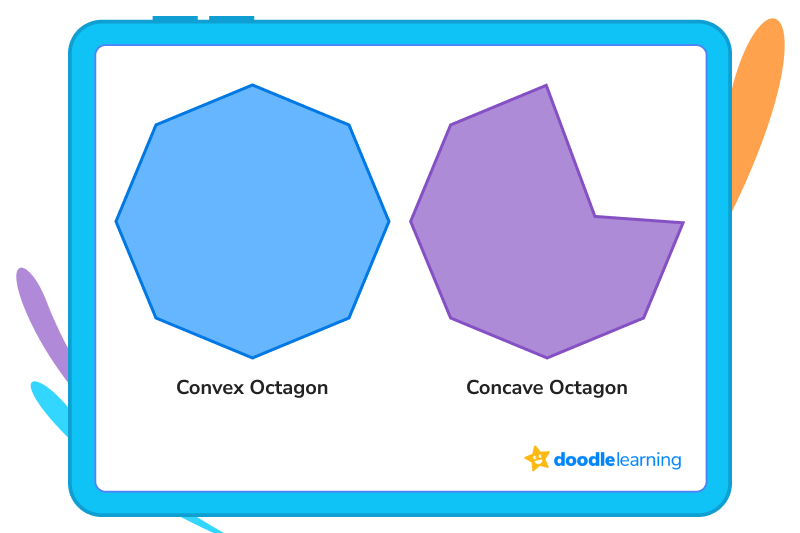## The perimeter of an octagonThe perimeter is the distance around the edge of a shape.

When you’re measuring the perimeter of an octagon, you will want to add together all 8 sides. Now, if each side is equal to one another and you know how to multiply, you can also multiply the length of one side by 8 to get the perimeter.

If you are dealing with an irregular octagon, you will probably have sides that have different lengths. This means you will need to look at each side and then add them all together.

### Examples of solving for perimeterNow, let’s take a look at how you would solve for perimeter for both a regular and irregular octagon.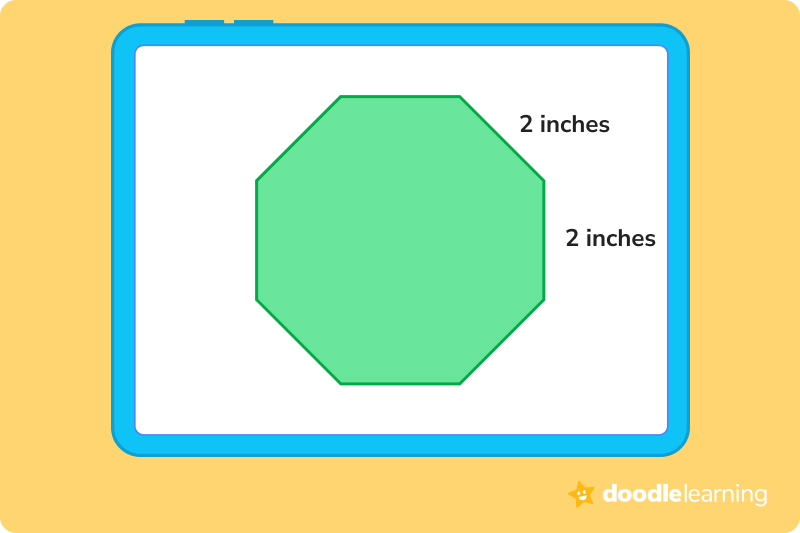This is a regular octagon. That means, we can use the equation P = 8a, where a is one side of the polygon.

So 8 × 2 = 16 inches

The perimeter is 16 inches.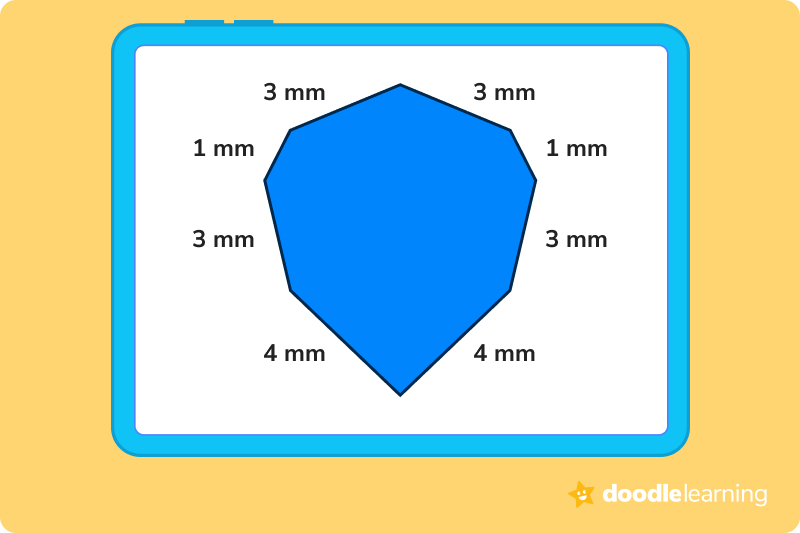This is an irregular octagon. That means we have to add the sides up.

1+1+3+3+3+3+4 = 18

The perimeter is 18mm.

Ready to give it a go?

Now that you’ve had a chance to learn all about octagons, let’s put your knowledge to the test. Answer the practice problems below and prove you’re an octagon wiz kid!

## Practice problems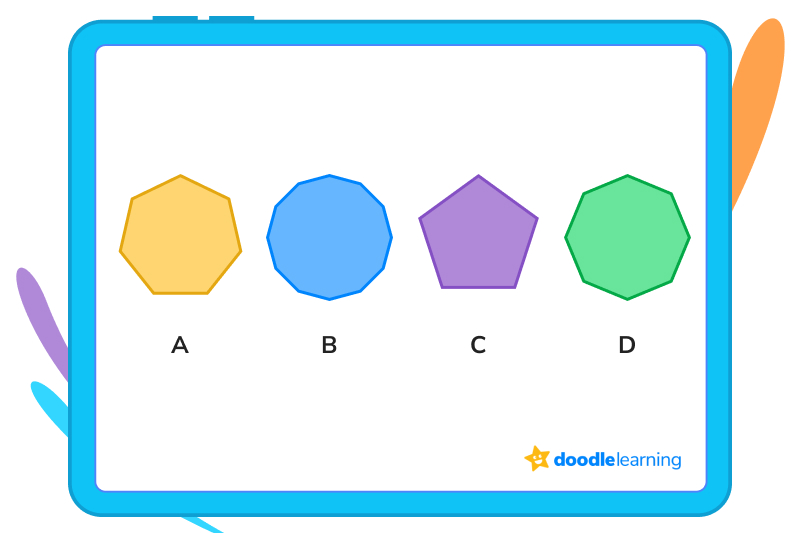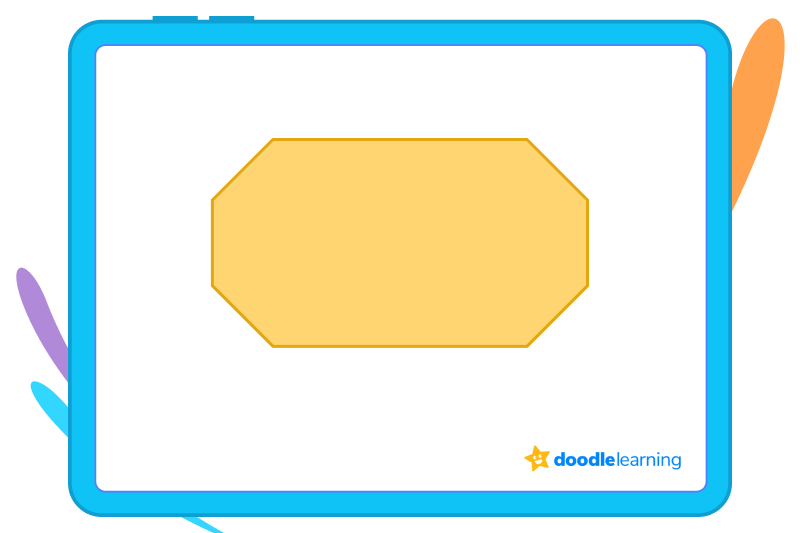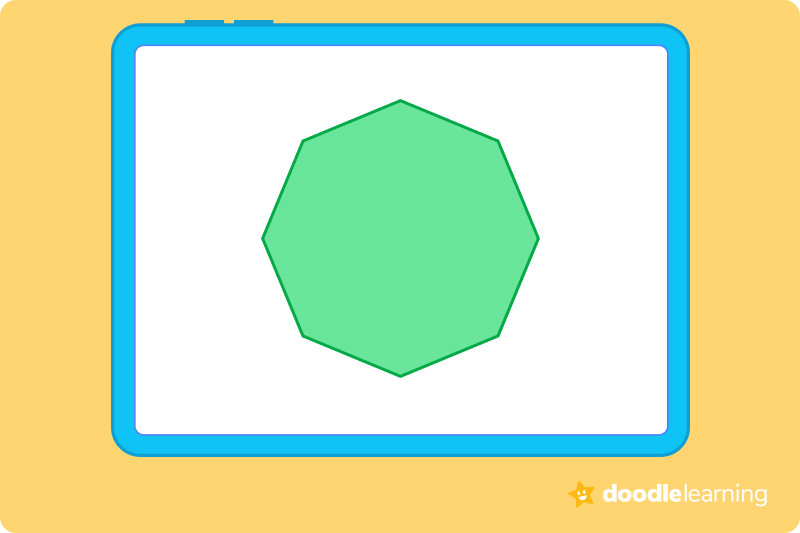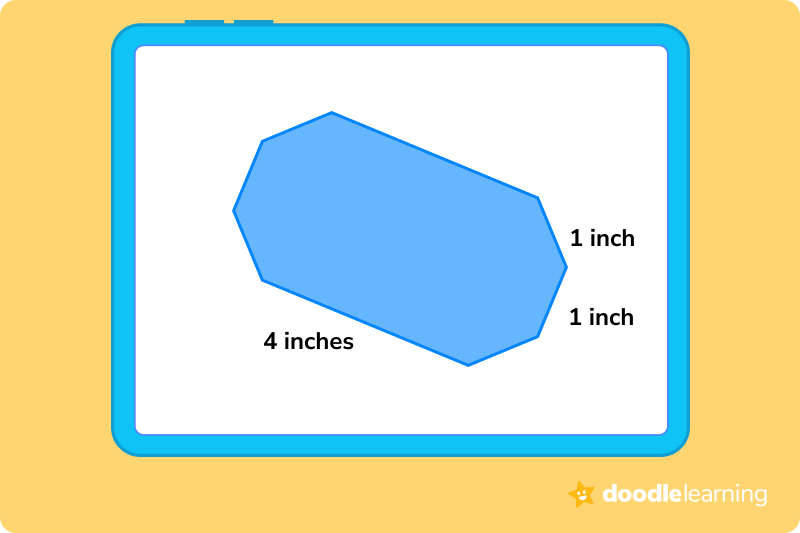## Parent Guide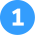How did we get here?

D is the only shape with 8 sides. An octagon must have 8 sides.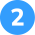How did we get here?

A regular octagon must have sides of equal length. This octagon has two sides that are longer than the other 6 sides. That makes it an irregular octagon.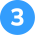How did we get here?

A concave octagon has some vertices that point inward. A convex octagon has no vertices that point inward. This octagon has no vertices that point inward. That means it is a convex octagon.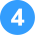How did we get here?

1. A regular octagon has equal sides.
2. So if each side is 8 inches, we multiply 8 by 8.
3. 8 inches × 8 inches = 64 inches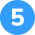How did we get here?

1. We know 6 of the sides equal 1 inch, so add those together, you get 6 inches.
2. The other two sides are each 4 inches, so add 4 to 4, you get 8 inches.
3. 8 inches + 6 inches = 14 inches.

## FAQs about math strategies for kidsWe understand that diving into new information can sometimes be overwhelming, and questions often arise. That’s why we’ve meticulously crafted these FAQs, based on real questions from students and parents. We’ve got you covered!

A basic octagon must have:

• 8 sides. They can be equal or unequal, but the magic number is 8.
• 8 angles. Each angle in an octagon measures 135 degrees.
• The sum of the angles equals 1080 degrees. To be a regular octagon, all the angles must add up to 1080 degrees.

Only if you are dealing with a regular octagon. In an irregular octagon, the sides may be different lengths, so you must add each side individually to get the proper measurement.

A regular octagon meets all the properties of an octagon (equal sides, equal angles, etc.) An irregular octagon is a closed shape with 8 sides, but it may not meet all the properties of a regular octagon.## Related Posts

Title 1

Title 2

Title 3

Lesson credits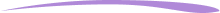Taylor Hartley

Taylor Hartley is an author and an English teacher based in Charlotte, North Carolina. When she's not writing, you can find her on the rowing machine or lost in a good novel.

Jill Padfield has 7 years of experience teaching high school mathematics, ranging from Alegra 1 to AP Calculas. She is currently working as a Business Analyst, working to improve services for Veterans while earning a masters degree in business administration.

Taylor Hartley

Taylor Hartley is an author and an English teacher based in Charlotte, North Carolina. When she's not writing, you can find her on the rowing machine or lost in a good novel.

Jill Padfield has 7 years of experience teaching high school mathematics, ranging from Alegra 1 to AP Calculas. She is currently working as a Business Analyst, working to improve services for Veterans while earning a masters degree in business administration.# Are you a parent, teacher or student?

Are you a parent or teacher?

## Hi there!

Book a chat with our team